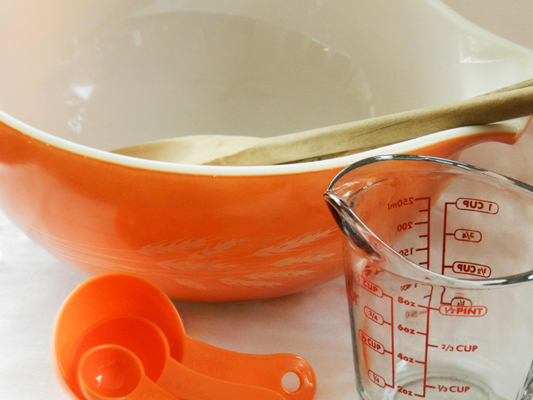# Measurement Equivalents

1
8432A handy online conversion table for both Imperial and Metric Measurements as well as a Common Equivalents Chart from the USDA Nutrient Data Laboratory.

## Measurement Equivalents

1 tablespoon (tbsp) = 3 teaspoons (tsp)

1/16 cup (c) = 1 tablespoon

1/8 cup = 2 tablespoons

1/6 cup = 2 tablespoons + 2 teaspoons

1/4 cup = 4 tablespoons

1/3 cup = 5 tablespoons + 1 teaspoon

3/8 cup = 6 tablespoons

1/2 cup = 8 tablespoons

2/3 cup = 10 tablespoons + 2 teaspoons

3/4 cup = 12 tablespoons

1 cup = 48 teaspoons

1 cup= 16 tablespoons

8 fluid ounces (fl oz) = 1 cup

1 pint (pt) = 2 cups

1 quart (qt) = 2 pints

4 cups = 1 quart

1 gallon (gal) = 4 quarts

16 ounces (oz) = 1 pound (lb)

1 milliliter (ml) = 1 cubic centimeter (cc)

1 inch (in) = 2.54 centimeters (cm)

## Metric Conversion Factors

Fluid Ounces (multiply by)  29.57  to get ( grams )

Ounces (dry) (multiply by)  28.35 to get ( grams )

Grams (multiply by) 0.0353 to get ( ounces )

Grams (multiply by) 0.0022 to get ( pounds )

Kilograms (multiply by) 2.21 to get  ( pounds )

Pounds (multiply by) 453.6 to get ( grams )

Pounds (multiply by) 0.4536 to get ( kilograms )

Quarts ( multiply by) 0.946 to get  ( liters )

Quarts (dry) (multiply by) 67.2 to get  ( cubic inches )

Quarts (liquid) (multiply by) 57.7 to get ( cubic inches )

Liters (multiply by) 1.0567 to get ( quarts )

Gallons (multiply by) 3, 785 to get ( cubic centimeters )

Gallons ( multiply by) 3,785 to get ( liters )

The Author:

Pioneerthinking.com – Ingredients for a Simple Life.

We Recommend

#### 1 COMMENT

1.Smithd658

Hi there, just became aware of your blog through Google, and found that it is really informative. Im going to watch out for brussels. Ill be grateful if you continue this in future. Lots of people will be benefited from your writing. Cheers!